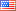# Revision history [back]

### Thickness of points in plots

How can I change the thickness of points in a graph?

In case it is not clear what I mean, consider the following program, illustrating Chebyshev's bias:

var('N') N=[] l=0 k=7 for p in prime_range(10^k+1): if (p==2): N.append(0)
else: if (Mod(p,4)==1): N.append(N[l]-1) l=l+1 else: N.append(N[l]+1) l=l+1 list_plot(N).show()

(Sorry, I cannot yet upload graphs, but you may quickly run this example and see that:) The dots seem too thick to rally appreciate any fine features of the graph. Is there a way to make the dots smaller (thinner)?2 formattingShashank1887 ●28 ●48 ●82

### Thickness of points in plots

How can I change the thickness of points in a graph?

In case it is not clear what I mean, consider the following program, illustrating Chebyshev's bias:

var('N')
N=[]
l=0
k=7
for p in prime_range(10^k+1):
if (p==2):
N.append(0)
else:
if (Mod(p,4)==1):
N.append(N[l]-1)
l=l+1
else:
N.append(N[l]+1)
l=l+1
list_plot(N).show()list_plot(N).show()


(Sorry, I cannot yet upload graphs, but you may quickly run this example and see that:) The dots seem too thick to rally appreciate any fine features of the graph. Is there a way to make the dots smaller (thinner)?